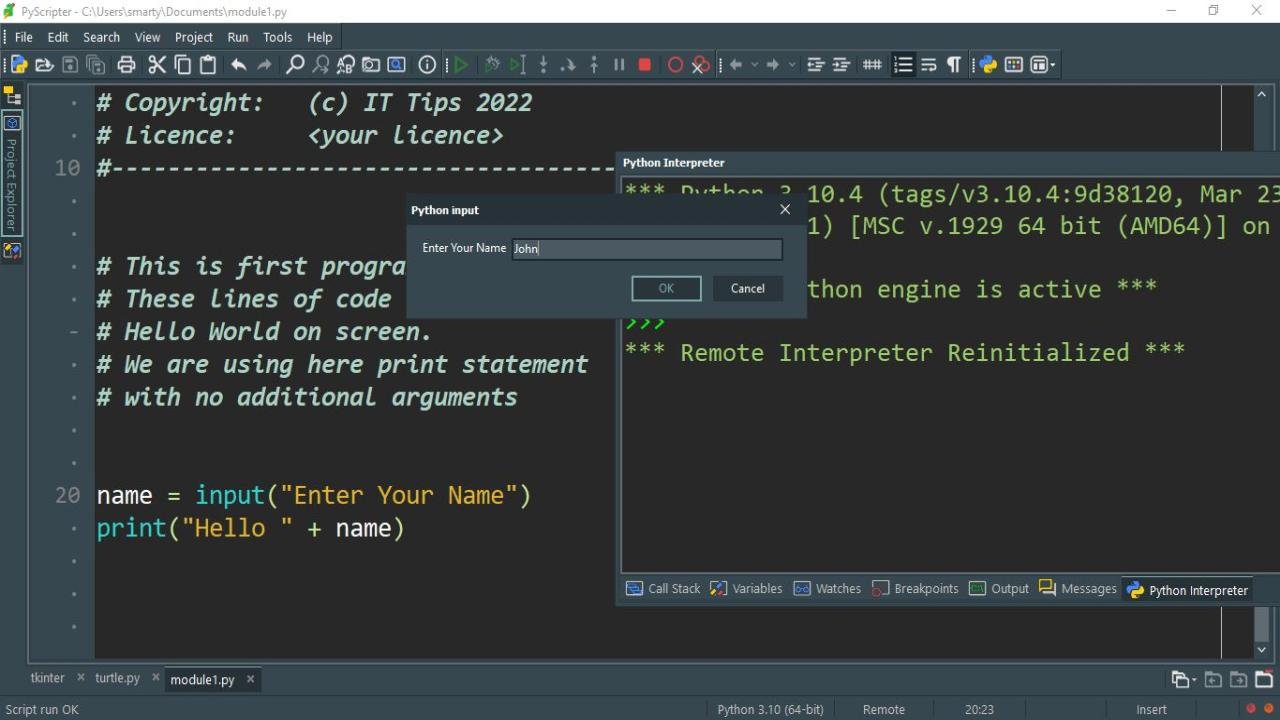# Python User Input

Getting user input and process the input data is an interactive feature that almost every programming language provide. Similarly by uing python language we can also take input in variables from user. This input is stored in variable and used in further processing.

## Python input function:

input() function is used in python for taking raw input. It prompts a message on screen and the user input save in variables.

### Syntax:

variable = input("prompt")

In python input() function variable and prompt is optional. We can also use input() function call without providing it prompt string. When input function is called a prompt dialog will be appear and input will be displayed in terminal.

## Python Raw Input:

In python programming input function takes input and return raw string that can be store in variable or can be displayed in terminal. Raw mean every input will be treated as string even user input integer or decimal values. So that if we are getting integer input then we have to use cast operator for processing input data.

### Example:

``````name = input("Enter Your Name")
print("Hello " + name)``````

In this example an input will be taken using python input() function. The input will be stored in name variable. After that this input is displayed using print function. The print() function will concatinate name with Hello string before displayed in output screen.

### Output:

After executing the code the following output will be displayed.## Python input string:

By default every input is handled as string input in python. So that for taking string input we not have to worry about input casting for processing. This string input can be used for further string manipulation easily.

### Example:

``````city = input("Enter Your City: ")
print("Welcome from " + city)``````

## Python input integer:

For processing integer type numeric data the input of input() function is required further casting into int. This can be done using int() function. After using this cast function the input will be treated as integer value.

### Example:

``````a = input("Enter First Number: ")
b = input("Enter Second Number: ")
sum = int(a)+ int(b)
print("Sum = " + str(sum))``````

## Difference between raw_input and input function:

In python both functions are used for taking input from user. raw_input is old function and was used in python 2 on the other hand input function was introduced in python 3.